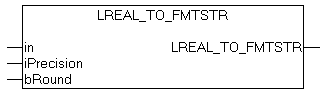# LREAL_TO_FMTSTRThe function converts and formats a floating-point number into a string variable with the following format: [ - ]dddd.dddd (dddd are decimal numbers). The number of digits before the decimal point depends on the value of the floating-point number. The number of digits after the decimal point depends on the required precision. The sign only appears for negative values. '#INF' is returned for infinite positive values, '-#INF' for infinite negative values. If the variable transferred has an illegal value (NaN, Not-a-Number), '#QNAN' or '-#QNAN' is returned. If the length of the formatted string exceeds the maximum permissible length of the resulting string, '#OVF' or '-#OVF' is returned.

## VAR_INPUT

``VAR_INPUT    in         : LREAL;    iPrecision : INT;    bRound     : BOOL;END_VAR``

in: Floating-point number that is to be converted and formatted.

iPrecision: Precision. The value determines the number of digits after the decimal point. With the minimum value (zero), no decimal places are displayed. The maximum value of iPrecision is limited by the number of digits before the decimal point and the maximum permissible length of the resulting string. If in = 0 and iPrecision = 0 then string '0' is returned.

bRound: If the bRound parameter is set, the formatted string is rounded to the respective number of decimal places (iPrecision). The following rule applies for rounding: If the decimal number after the last required decimal place is >= 5, the value is rounded up, otherwise not.

Example 1:

0.46523 is to be converted into a string with two decimal places and rounded.

``sOut := LREAL_TO_FMTSTR( 0.46523, 2, TRUE );``

The result is: '0.47';

Example: 2The maximum number of significant digits for LREAL variables is limited to 15.Due to the internal representation of floating-point numbers and rounding errors during conversion, the resulting string may not precisely correspond to the value of the in variable.
``PROGRAM MAINVAR    double : LREAL;    s1     : STRING;    s2     : STRING;    s3     : STRING;    s4     : STRING;END_VAR``
``double := 0.5;s1     := LREAL_TO_FMTSTR( double, 25, FALSE );s2     := LREAL_TO_FMTSTR( double, 2, FALSE );s3     := LREAL_TO_FMTSTR( double, 0, TRUE );s4     := LREAL_TO_FMTSTR( double, 2, TRUE );``

The result is:

s1 = '0.4999999999999999756000000' This is how double variables are represented internally. This number is used as the starting point for the rounding operation.

s2 = '0.49'

Rounding leads to the following results:

s3 = '0'

s4 = '0.50'

## Requirements

Development environment

Target platform

PLC libraries to be integrated (category group)

TwinCAT v3.1.0

PC or CX (x86, x64, ARM)

Tc2_Utilities (System)